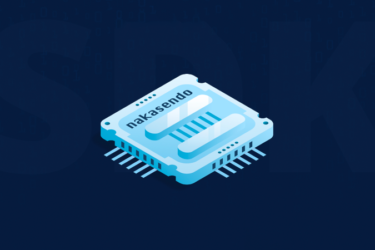# Edge Cases: Part 2.1 — Nodes & Edges

Read the full post and the rest of the series on the nChain Medium account here.

Welcome back to Edge Cases, the Metanet blog series.

In the last post, we introduced several concepts underpinning directed acyclic graphs (DAGs), and looked at how they are related to the Metanet protocol. If you’re just joining us, you can find the start of the series here. In today’s post, we are going to look at exactly how the Metanet protocol can be used to create on-chain DAG structures that can facilitate a peer-to-peer value network on Bitcoin SV.

## Part 2.1 — Node and Edge Structure

In part 2 of the series, we met a little bit of graph theory, and I introduced some of its foundational concepts that allowed us to describe DAGs. As a brief recap, the salient points are:

• graphs — structures comprising nodes and edges;
• directionality — the direction of an edge in a directed graph;
• DAGs — structures that are graphs, have directed edges and are acyclic;
• in-degree — the number of edges pointing into a node; and
• out-degree — the number of edges pointing out of a node.

These simple facts allowed us to build up an understanding of directed acyclic graph structures in principle. From here, we were able to apply our basic comprehension of their related concepts to help us describe three graphs:

1. the Block DAG;
2. the Transaction DAG; and
3. the Metanet DAG.

We observed that, for a proof-of-work blockchain such as Bitcoin SV, the blocks indeed form a simple, linear DAG, which we named the ‘Block DAG.’ Similarly, we found that the transactions also form a DAG, albeit a much more complex one, which we termed the ‘Transaction DAG.’

Finally, we introduced the notion of the Metanet graph, which is also a directed acyclic graph. We have discussed how the Metanet protocoldefines a set of rules that allow us to create the Metanet graph, analogously to how the underlying blockchain protocol defines the set of rules that allow us to create both the Block and the Transaction DAGs.

In today’s post, we will look in detail at how the Metanet protocol achieves on-chain graph structures, built natively and exclusively on the Bitcoin SV blockchain, before looking to formalise the rule set of the Metanet protocol, and explore the features and properties of the graph structures its rules facilitate.

### Metanet Nodes

In accordance with the technical summary, a Metanet node is simply a transactionthat follows the rule set of the Metanet protocol.

Nodes are transactions.

As we will learn, the rules governing what a Metanet node actually looks like are highly unrestrictive, which means that Metanet nodes can take many different shapes, sizes and forms. Indeed, any transaction which follows the very simple Metanet node format, as a skeletal outline, is considered a Metanet node. So, what is this format?

• The transaction has at least one OP_RETURN output;
• The OP_RETURN payload includes: (i) the Metanet flag, (ii) a node address ‘P(node),’ and (iii) a parent transaction ID ‘TxID(parent).’
• The transaction contains an input signed by a parent node, denoted by ‘SigP(parent),’ if that node does indeed have a parent.

What may sound like a lot of requirements when written down is often much clearer when expressed diagrammatically in Bitcoin. The simplest outline structure of a Metanet node, which satisfies all of the criteria above, is shown in the diagram below:

A Metanet node transaction.

We can see that the given example is a very simple transaction in reality. Taken at face value, it just looks like a fairly standard OP_RETURN transaction with three elements in its OP_RETURN data payload. The key difference between Metanet nodes and such transactions, which we are highly familiar with and seeing already in Bitcoin SV, is that the Metanet protocol imbues meaning to the components of such transactions.

What exactly do I mean here?

The transaction above, showing the skeleton for a Metanet node, can be viewed as comprising six elements:

• TxID(node);
• SigP(parent);
• P(parent);
• Metanet flag;
• P(node); and
• TxID(parent).

The first three of these elements, the TxID(node), SigP(parent), and P(parent), would be present as part of any typical transaction. Moreover, in a typical OP_RETURN output payload, we would expect at least one element, and so it is not unreasonable to say that the remaining three elements, the Metanet flag, P(node), and TxID(parent), may also be present in any typical OP_RETURN payload.

The core of the Metanet protocol is that we ascribe very specific meaning to each of these six elements, where, in the traditional case, only a few of them may have intrinsic meaning. These specific meanings applied by the Metanet protocol are:

• TxID(node) — the unique transaction ID of the node;
• SigP(parent) — a signature created by the parent of the node;
• P(parent) — the address defining the parent of the node;
• Metanet flag — a protocol prefix signalling a Metanet transaction;
• P(node) — the address defining the node; and
• TxID(parent) — the unique transaction ID of the parent of the node.

I want to reiterate once more here the crucial aspect is that we have ascribed meaning to all six of these basic elements of the node transaction. The Metanet protocol thereby stands in contrast to existing OP_RETURN protocols, which tend to ignore the utility of the input signature and only stipulate a specific meaning for elements in the OP_RETURN payload.